• 用scikit-learn进行LDA降维 　在线性判别分析LDA原理总结中，我们对LDA降维的原理做了总结，这里我们就对scikit-learn中LDA降维使用做一个总结。 1.对scikit-learn中LDA类概述 　在scikit-learn中， LDA类是...
用scikit-learn进行LDA降维
在线性判别分析LDA原理总结中，我们对LDA降维的原理做了总结，这里我们就对scikit-learn中LDA的降维使用做一个总结。
1. 对scikit-learn中LDA类概述
在scikit-learn中， LDA类是sklearn.discriminant_analysis.LinearDiscriminantAnalysis。那既可以用于分类又可以用于降维。当然，应用场景最多的还是降维。和PCA类似，LDA降维基本也不用调参，只需要指定降维到的维数即可。
2. LinearDiscriminantAnalysis类概述
我们这里对LinearDiscriminantAnalysis类的参数做一个基本的总结。
1）solver : 即求LDA超平面特征矩阵使用的方法。可以选择的方法有奇异值分解"svd"，最小二乘"lsqr"和特征分解"eigen"。一般来说特征数非常多的时候推荐使用svd，而特征数不多的时候推荐使用eigen。主要注意的是，如果使用svd，则不能指定正则化参数shrinkage进行正则化。默认值是svd
2）shrinkage：正则化参数，可以增强LDA分类的泛化能力。如果仅仅只是为了降维，则一般可以忽略这个参数。默认是None，即不进行正则化。可以选择"auto",让算法自己决定是否正则化。当然我们也可以选择不同的[0,1]之间的值进行交叉验证调参。注意shrinkage只在solver为最小二乘"lsqr"和特征分解"eigen"时有效。
3）priors ：类别权重，可以在做分类模型时指定不同类别的权重，进而影响分类模型建立。降维时一般不需要关注这个参数。
4）n_components：即我们进行LDA降维时降到的维数。在降维时需要输入这个参数。注意只能为[1,类别数-1)范围之间的整数。如果我们不是用于降维，则这个值可以用默认的None。
从上面的描述可以看出，如果我们只是为了降维，则只需要输入n_components,注意这个值必须小于“类别数-1”。PCA没有这个限制。
3. LinearDiscriminantAnalysis降维实例
在LDA的原理篇我们讲到，PCA和LDA都可以用于降维。两者没有绝对的优劣之分，使用两者的原则实际取决于数据的分布。由于LDA可以利用类别信息，因此某些时候比完全无监督的PCA会更好。下面我们举一个LDA降维可能更优的例子。
完整代码参加我的github: https://github.com/ljpzzz/machinelearning/blob/master/classic-machine-learning/lda.ipynb

我们首先生成三类三维特征的数据，代码如下：
import numpy as np
import matplotlib.pyplot as plt
from mpl_toolkits.mplot3d import Axes3D
%matplotlib inline
from sklearn.datasets.samples_generator import make_classification
X, y = make_classification(n_samples=1000, n_features=3, n_redundant=0, n_classes=3, n_informative=2,
n_clusters_per_class=1,class_sep =0.5, random_state =10)
fig = plt.figure()
ax = Axes3D(fig, rect=[0, 0, 1, 1], elev=30, azim=20)
ax.scatter(X[:, 0], X[:, 1], X[:, 2],marker='o',c=y)
我们看看最初的三维数据的分布情况：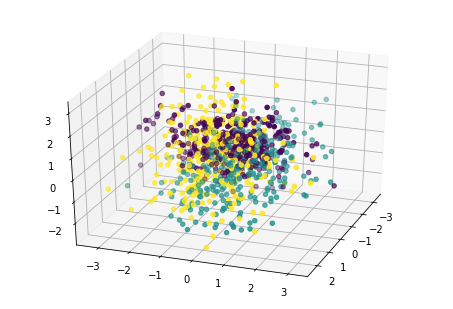首先我们看看使用PCA降维到二维的情况，注意PCA无法使用类别信息来降维，代码如下：
from sklearn.decomposition import PCA
pca = PCA(n_components=2)
pca.fit(X)
print pca.explained_variance_ratio_
print pca.explained_variance_
X_new = pca.transform(X)
plt.scatter(X_new[:, 0], X_new[:, 1],marker='o',c=y)
plt.show()
在输出中，PCA找到的两个主成分方差比和方差如下：
[ 0.43377069  0.3716351 ]
[ 1.20962365  1.03635081]
输出的降维效果图如下: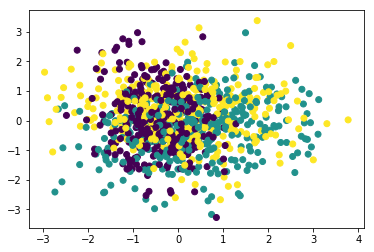由于PCA没有利用类别信息，我们可以看到降维后，样本特征和类别的信息关联几乎完全丢失。
现在我们再看看使用LDA的效果，代码如下：
from sklearn.discriminant_analysis import LinearDiscriminantAnalysis
lda = LinearDiscriminantAnalysis(n_components=2)
lda.fit(X,y)
X_new = lda.transform(X)
plt.scatter(X_new[:, 0], X_new[:, 1],marker='o',c=y)
plt.show()
输出的效果图如下：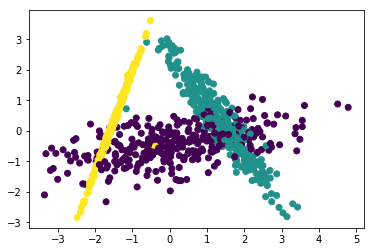可以看出降维后样本特征和类别信息之间的关系得以保留。
一般来说，如果我们的数据是有类别标签的，那么优先选择LDA去尝试降维；当然也可以使用PCA做很小幅度的降维去消去噪声，然后再使用LDA降维。如果没有类别标签，那么肯定PCA是最先考虑的一个选择了。

（欢迎转载，转载请注明出处。欢迎沟通交流： liujianping-ok@163.com） 
展开全文sklearn 机器学习
• ↑↑点击上方蓝字，更多惊喜来源 |DataWhale网上关于各种降维算法的资料参差不齐，同时大部分不提供源代码。这里有个 GitHub 项目整理了使用 Python 实现了 11 种经...

↑↑点击上方蓝字，更多惊喜来源 | DataWhale
网上关于各种降维算法的资料参差不齐，同时大部分不提供源代码。这里有个 GitHub 项目整理了使用 Python 实现了 11 种经典的数据抽取(数据降维)算法，包括：PCA、LDA、MDS、LLE、TSNE 等，并附有相关资料、展示效果;非常适合机器学习初学者和刚刚入坑数据挖掘的小伙伴。
一、为什么要进行数据降维?
所谓降维，即用一组个数为 d 的向量 Zi 来代表个数为 D 的向量 Xi 所包含的有用信息，其中 d<D，通俗来讲，即将高维度下降至低维度；将高维数据下降为低维数据。
通常，我们会发现大部分数据集的维度都会高达成百乃至上千，而经典的 MNIST，其维度都是 64。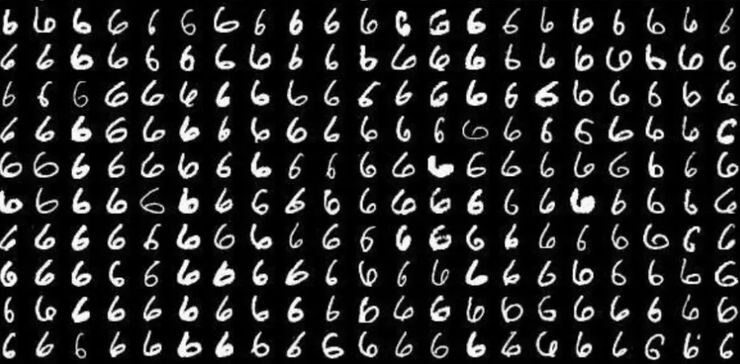MNIST 手写数字数据集
但在实际应用中，我们所用到的有用信息却并不需要那么高的维度，而且每增加一维所需的样本个数呈指数级增长，这可能会直接带来极大的「维数灾难」;而数据降维就可以实现：
使得数据集更易使用确保变量之间彼此独立降低算法计算运算成本
去除噪音一旦我们能够正确处理这些信息，正确有效地进行降维，这将大大有助于减少计算量，进而提高机器运作效率。而数据降维，也常应用于文本处理、人脸识别、图片识别、自然语言处理等领域。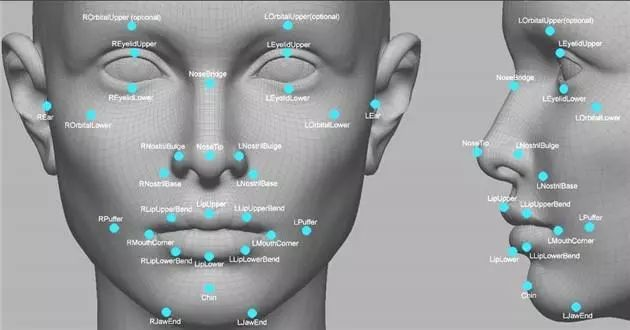二、数据降维原理
往往高维空间的数据会出现分布稀疏的情况，所以在降维处理的过程中，我们通常会做一些数据删减，这些数据包括了冗余的数据、无效信息、重复表达内容等。
例如：现有一张 1024*1024 的图，除去中心 50*50 的区域其它位置均为零值，这些为零的信息就可以归为无用信息;而对于对称图形而言，对称部分的信息则可以归为重复信息。因此，大部分经典降维技术也是基于这一内容而展开，其中降维方法又分为线性和非线性降维，非线性降维又分为基于核函数和基于特征值的方法。
线性降维方法：PCA 、ICA LDA、LFA、LPP(LE 的线性表示)非线性降维方法：
基于核函数的非线性降维方法——KPCA 、KICA、KDA基于特征值的非线性降维方法(流型学习)——ISOMAP、LLE、LE、LPP、LTSA、MVU
哈尔滨工业大学计算机技术专业的在读硕士生 Heucoder 则整理了 PCA、KPCA、LDA、MDS、ISOMAP、LLE、TSNE、AutoEncoder、FastICA、SVD、LE、LPP 共 12 种经典的降维算法，并提供了相关资料、代码以及展示，下面将主要以 PCA 算法为例介绍降维算法具体操作。
三、主成分分析(PCA)降维算
PCA 是一种基于从高维空间映射到低维空间的映射方法，也是最基础的无监督降维算法，其目标是向数据变化最大的方向投影，或者说向重构误差最小化的方向投影。它由 Karl Pearson 在 1901 年提出，属于线性降维方法。与 PCA 相关的原理通常被称为最大方差理论或最小误差理论。这两者目标一致，但过程侧重点则不同。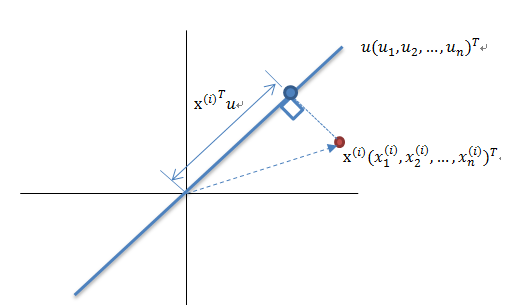最大方差理论降维原理
将一组 N 维向量降为 K 维(K 大于 0，小于 N)，其目标是选择 K 个单位正交基，各字段两两间 COV(X,Y) 为 0，而字段的方差则尽可能大。因此，最大方差即使得投影数据的方差被最大化，在这过程中，我们需要找到数据集 Xmxn 的最佳的投影空间 Wnxk、协方差矩阵等，其算法流程为：
算法输入：数据集 Xmxn;按列计算数据集 X 的均值 Xmean，然后令 Xnew=X−Xmean;求解矩阵 Xnew 的协方差矩阵，并将其记为 Cov;计算协方差矩阵 COV 的特征值和相应的特征向量;将特征值按照从大到小的排序，选择其中最大的 k 个，然后将其对应的 k 个特征向量分别作为列向量组成特征向量矩阵 Wnxk;计算 XnewW，即将数据集 Xnew 投影到选取的特征向量上，这样就得到了我们需要的已经降维的数据集 XnewW。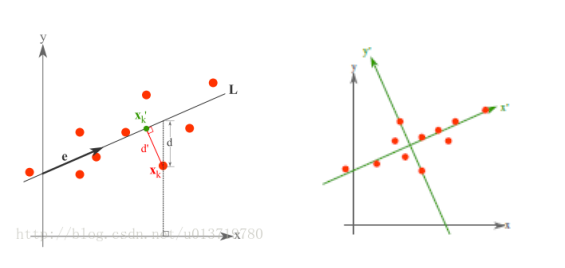最小误差理论降维原理
而最小误差则是使得平均投影代价最小的线性投影，这一过程中，我们则需要找到的是平方错误评价函数 J0(x0) 等参数。
详细步骤可参考《从零开始实现主成分分析 (PCA) 算法》：
https://blog.csdn.net/u013719780/article/details/78352262
主成分分析(PCA)代码实现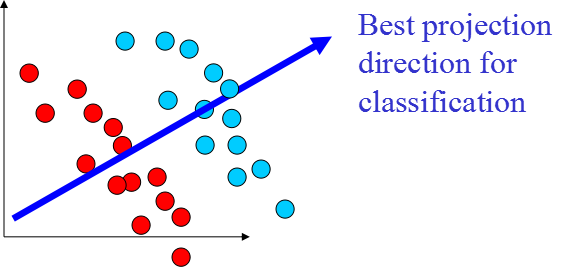关于 PCA 算法的代码如下：
from __future__ import print_function
from sklearn import datasets
import matplotlib.pyplot as plt
import matplotlib.cm as cmx
import matplotlib.colors as colors
import numpy as np
%matplotlib inline

def shuffle_data(X, y, seed=None):
if seed:
np.random.seed(seed)

idx = np.arange(X.shape)
np.random.shuffle(idx)

return X[idx], y[idx]

# 正规化数据集 X
def normalize(X, axis=-1, p=2):
lp_norm = np.atleast_1d(np.linalg.norm(X, p, axis))
lp_norm[lp_norm == 0] = 1
return X / np.expand_dims(lp_norm, axis)

# 标准化数据集 X
def standardize(X):
X_std = np.zeros(X.shape)
mean = X.mean(axis=0)
std = X.std(axis=0)

# 做除法运算时请永远记住分母不能等于 0 的情形
# X_std = (X - X.mean(axis=0)) / X.std(axis=0)
for col in range(np.shape(X)):
if std[col]:
X_std[:, col] = (X_std[:, col] - mean[col]) / std[col]
return X_std

# 划分数据集为训练集和测试集
def train_test_split(X, y, test_size=0.2, shuffle=True, seed=None):
if shuffle:
X, y = shuffle_data(X, y, seed)
n_train_samples = int(X.shape * (1-test_size))
x_train, x_test = X[:n_train_samples], X[n_train_samples:]
y_train, y_test = y[:n_train_samples], y[n_train_samples:]

return x_train, x_test, y_train, y_test

# 计算矩阵 X 的协方差矩阵
def calculate_covariance_matrix(X, Y=np.empty((0,0))):
if not Y.any():
Y = X
n_samples = np.shape(X)
covariance_matrix = (1 / (n_samples-1)) * (X - X.mean(axis=0)).T.dot(Y - Y.mean(axis=0))
return np.array(covariance_matrix, dtype=float)

# 计算数据集 X 每列的方差
def calculate_variance(X):
n_samples = np.shape(X)
variance = (1 / n_samples) * np.diag((X - X.mean(axis=0)).T.dot(X - X.mean(axis=0)))
return variance

# 计算数据集 X 每列的标准差
def calculate_std_dev(X):
std_dev = np.sqrt(calculate_variance(X))
return std_dev

# 计算相关系数矩阵
def calculate_correlation_matrix(X, Y=np.empty()):
# 先计算协方差矩阵
covariance_matrix = calculate_covariance_matrix(X, Y)
# 计算 X, Y 的标准差
std_dev_X = np.expand_dims(calculate_std_dev(X), 1)
std_dev_y = np.expand_dims(calculate_std_dev(Y), 1)
correlation_matrix = np.divide(covariance_matrix, std_dev_X.dot(std_dev_y.T))

return np.array(correlation_matrix, dtype=float)

class PCA():
"""
主成份分析算法 PCA，非监督学习算法.
"""
def __init__(self):
self.eigen_values = None
self.eigen_vectors = None
self.k = 2

def transform(self, X):
"""
将原始数据集 X 通过 PCA 进行降维
"""
covariance = calculate_covariance_matrix(X)

# 求解特征值和特征向量
self.eigen_values, self.eigen_vectors = np.linalg.eig(covariance)

# 将特征值从大到小进行排序，注意特征向量是按列排的，即 self.eigen_vectors 第 k 列是 self.eigen_values 中第 k 个特征值对应的特征向量
idx = self.eigen_values.argsort()[::-1]
eigenvalues = self.eigen_values[idx][:self.k]
eigenvectors = self.eigen_vectors[:, idx][:, :self.k]

# 将原始数据集 X 映射到低维空间
X_transformed = X.dot(eigenvectors)

return X_transformed

def main():
X = data.data
y = data.target

# 将数据集 X 映射到低维空间
X_trans = PCA().transform(X)

x1 = X_trans[:, 0]
x2 = X_trans[:, 1]

cmap = plt.get_cmap('viridis')
colors = [cmap(i) for i in np.linspace(0, 1, len(np.unique(y)))]

class_distr = []
# Plot the different class distributions
for i, l in enumerate(np.unique(y)):
_x1 = x1[y == l]
_x2 = x2[y == l]
_y = y[y == l]
class_distr.append(plt.scatter(_x1, _x2, color=colors[i]))

plt.legend(class_distr, y, loc=1)

# Axis labels
plt.xlabel('Principal Component 1')
plt.ylabel('Principal Component 2')
plt.show()

if __name__ == "__main__":
main()
最终，我们将得到降维结果如下。其中，如果得到当特征数 (D) 远大于样本数 (N) 时，可以使用一点小技巧实现 PCA 算法的复杂度转换。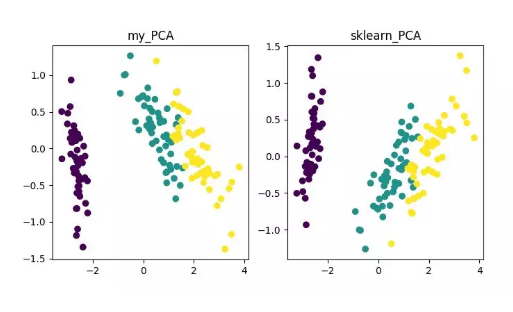PCA 降维算法展示
当然，这一算法虽然经典且较为常用，其不足之处也非常明显。它可以很好的解除线性相关，但是面对高阶相关性时，效果则较差;同时，PCA 实现的前提是假设数据各主特征是分布在正交方向上，因此对于在非正交方向上存在几个方差较大的方向，PCA 的效果也会大打折扣。
四、其它降维算法及代码地址
KPCA(kernel PCA)
KPCA 是核技术与 PCA 结合的产物，它与 PCA 主要差别在于计算协方差矩阵时使用了核函数，即是经过核函数映射之后的协方差矩阵。
引入核函数可以很好的解决非线性数据映射问题。kPCA 可以将非线性数据映射到高维空间，在高维空间下使用标准 PCA 将其映射到另一个低维空间。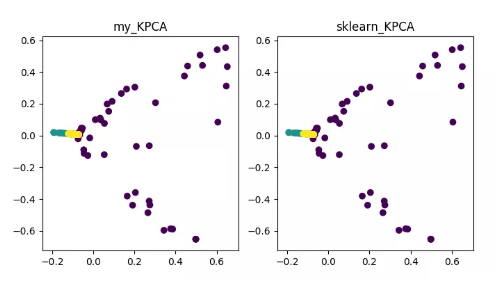KPCA 降维算法展示
详细内容可参见 《Python 机器学习》之特征抽取——kPCA：
https://blog.csdn.net/weixin_40604987/article/details/79632888
代码地址：
https://github.com/heucoder/dimensionality_reduction_alo_codes/blob/master/codes/PCA/KPCA.py
LDA(Linear Discriminant Analysis)
LDA 是一种可作为特征抽取的技术，其目标是向最大化类间差异，最小化类内差异的方向投影，以利于分类等任务即将不同类的样本有效的分开。LDA 可以提高数据分析过程中的计算效率，对于未能正则化的模型，可以降低维度灾难带来的过拟合。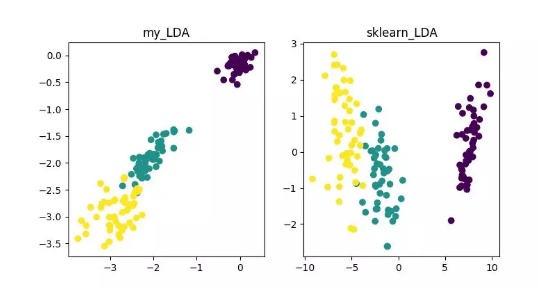LDA 降维算法展示
详细内容可参见《数据降维—线性判别分析(LDA)》：
https://blog.csdn.net/ChenVast/article/details/79227945
代码地址：
https://github.com/heucoder/dimensionality_reduction_alo_codes/tree/master/codes/LDA
MDS(multidimensional scaling)
MDS 即多维标度分析，它是一种通过直观空间图表示研究对象的感知和偏好的传统降维方法。该方法会计算任意两个样本点之间的距离，使得投影到低维空间之后能够保持这种相对距离从而实现投影。
由于 sklearn 中 MDS 是采用迭代优化方式，下面实现了迭代和非迭代的两种。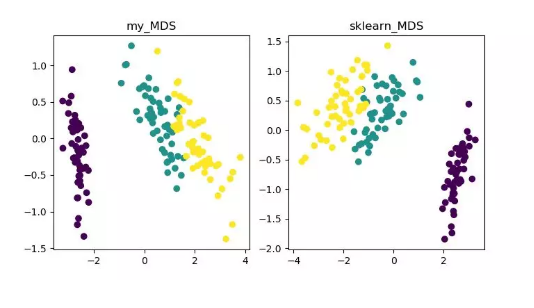MDS 降维算法展示
详细内容可参见《MDS 算法》
https://blog.csdn.net/zhangweiguo_717/article/details/69663452
代码地址：
https://github.com/heucoder/dimensionality_reduction_alo_codes/tree/master/codes/MDS
ISOMAP
Isomap 即等度量映射算法，该算法可以很好地解决 MDS 算法在非线性结构数据集上的弊端。
MDS 算法是保持降维后的样本间距离不变，Isomap 算法则引进了邻域图，样本只与其相邻的样本连接，计算出近邻点之间的距离，然后在此基础上进行降维保距。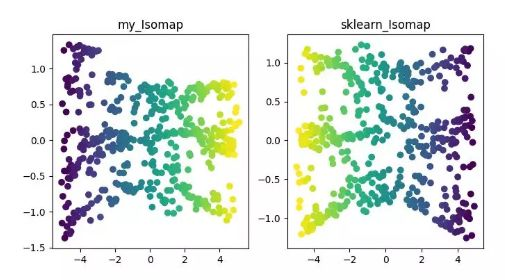ISOMAP 降维算法展示
详细内容可参见《Isomap》
https://blog.csdn.net/zhangweiguo_717/article/details/69802312
代码地址：
https://github.com/heucoder/dimensionality_reduction_alo_codes/tree/master/codes/ISOMAP
LLE(locally linear embedding)
LLE(locally linear embedding)LLE 即局部线性嵌入算法，它是一种非线性降维算法。该算法核心思想为每个点可以由与它相邻的多个点的线性组合而近似重构，然后将高维数据投影到低维空间中，使其保持数据点之间的局部线性重构关系，即有相同的重构系数。在处理所谓的流形降维的时候，效果比 PCA 要好很多。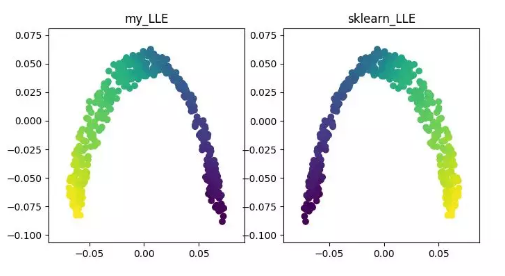LLE 降维算法展示
详细内容可参见《LLE 原理及推导过程》
https://blog.csdn.net/scott198510/article/details/76099630
代码地址：
https://github.com/heucoder/dimensionality_reduction_alo_codes/tree/master/codes/LLE
t-SNE
t-SNE 也是一种非线性降维算法，非常适用于高维数据降维到 2 维或者 3 维进行可视化。它是一种以数据原有的趋势为基础，重建其在低纬度(二维或三维)下数据趋势的无监督机器学习算法。
下面的结果展示参考了源代码，同时也可用 tensorflow 实现(无需手动更新参数)。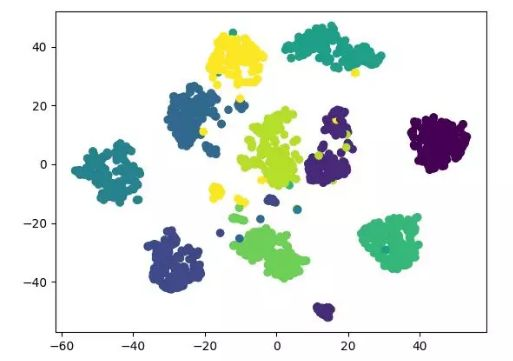t-SNE 降维算法展示
详细内容可参见《t-SNE 使用过程中的一些坑》：
http://bindog.github.io/blog/2018/07/31/t-sne-tips/
代码地址：
https://github.com/heucoder/dimensionality_reduction_alo_codes/tree/master/codes/T-SNE
LE(Laplacian Eigenmaps)
LE 即拉普拉斯特征映射，它与 LLE 算法有些相似，也是以局部的角度去构建数据之间的关系。它的直观思想是希望相互间有关系的点(在图中相连的点)在降维后的空间中尽可能的靠近;以这种方式，可以得到一个能反映流形的几何结构的解。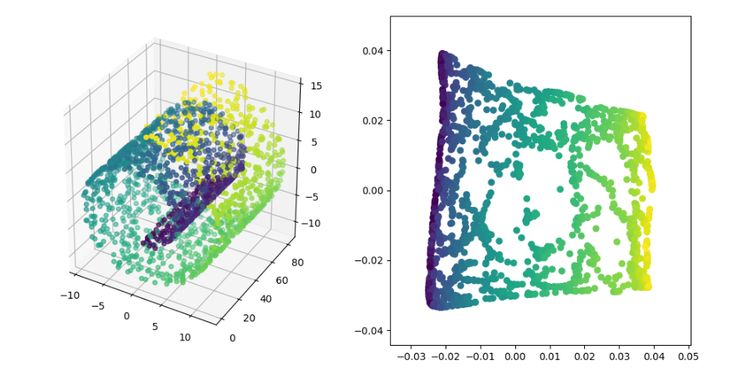LE 降维算法展示
详细内容可参见《拉普拉斯特征图降维及其 python 实现》：
https://blog.csdn.net/HUSTLX/article/details/50850342
代码地址：
https://github.com/heucoder/dimensionality_reduction_alo_codes/tree/master/codes/LE
LPP(Locality Preserving Projections)
LPP 即局部保留投影算法，其思路和拉普拉斯特征映射类似，核心思想为通过最好的保持一个数据集的邻居结构信息来构造投影映射，但 LPP 不同于 LE 的直接得到投影结果，它需要求解投影矩阵。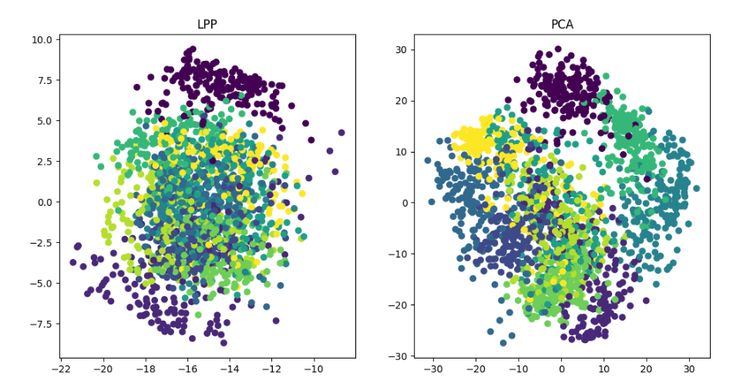LPP 降维算法展示
详情请参见《局部保留投影算法 (LPP) 详解》：
https://blog.csdn.net/qq_39187538/article/details/90402961
代码地址：
https://github.com/heucoder/dimensionality_reduction_alo_codes/tree/master/codes/LPP
*《dimensionality_reduction_alo_codes》项目作者简介
Heucoder，目前是哈尔滨工业大学计算机技术在读硕士生，主要活跃于互联网领域，知乎昵称为「超爱学习」，其 github 主页地址为：https://github.com/heucoder。
Github 项目地址:
https://github.com/heucoder/dimensionality_reduction_alo_codes




展开全文• LDA和PCA降维的区别
这里主要介绍LDA和PCA的区别：
相同点：
1）两者均可以对数据进行降维。
2）两者在降维时均使用了矩阵特征分解的思想。
3）两者都假设数据符合高斯分布。
不同点：
1）LDA是有监督的降维方法，而PCA是无监督的降维方法
2）LDA降维最多降到类别数k-1的维数，而PCA没有这个限制。
3）LDA除了可以用于降维，还可以用于分类。
4）LDA选择分类性能最好的投影方向，而PCA选择样本点投影具有最大方差的方向。
LDA和PCA所用库：
import numpy as np
import matplotlib.pyplot as plt
from mpl_toolkits.mplot3d import Axes3D  # 用来绘制3D图
from sklearn.decomposition import PCA
from sklearn.discriminant_analysis import LinearDiscriminantAnalysis as LDA

lda代码如下：
def lda_():
x = data[:, 1:]
y = data[:, 0].astype(int)
y = np.array(y).reshape(-1, 1)
lda1 = LDA(n_components=2)
x_r = lda1.fit(x, y).transform(x)
fig2 = plt.figure()
ax2.scatter(x_r[:, 0], x_r[:, 1], marker='o', c=y)

fig2.savefig('picture/f_lda.png', bbox_inches='tight')

由于lda只可以最多降维到k-1类特征，我的数据集只有3类，所以我设置n_components=2，最后结果如下：pca代码如下：
def pca_():
x = datamat[:, 1:]
y = datamat[:, 0].astype(int)
y = np.array(y).reshape(-1, 1)
datamat = x
pca1 = PCA(n_components=2)  # n_components表示保留主成分个数
pca1.fit(datamat)  # 用数据datamet来训练PCA模型
lowdata = pca1.transform(datamat)  # 对数据归一化
"""
fig = plt.figure()
ax1 = Axes3D(fig)
#ax1.scatter(reconmat1[:, 0], reconmat1[:, 1], reconmat1[:, 2], cmap='Blues')
#ax1.scatter(reconmat4[:, 0], reconmat4[:, 1], reconmat4[:, 2], cmap='red')
#ax1.scatter(reconmat7[:, 0], reconmat7[:, 1], reconmat7[:, 2], cmap='#bcbd22')
fig.savefig('picture/f3D.png', bbox_inches='tight')
"""

fig1 = plt.figure()
ax.scatter(lowdata[:, 0], lowdata[:, 1], marker='o', c=y)
# fig1.show()
fig1.savefig('picture/f2D.png', bbox_inches='tight')可以看出我这里的实验，使用LDA比PCA效果要好一点，对于数据有类别的，建议使用LDA进行降维。
展开全文计算机视觉
• 目录简介算法流程基于python sklearn库的LDA例程 简介 线性判别分析（Linear Discriminate Analysis, LDA）通过正交变换将一组可能存在相关性的变量降维变量，目标是将高维数据投影至低维后，同类的数据之间距离尽...


目录
简介算法流程基于python sklearn库的LDA例程

简介
线性判别分析（Linear Discriminate Analysis, LDA）通过正交变换将一组可能存在相关性的变量降维变量，目标是将高维数据投影至低维后，同类的数据之间距离尽可能近、不同类数据之间距离尽可能远。
应用场景： 对于拟合、分类算法，可以利用主成分分析对输入数据实现降维，去除冗余数据可以提高计算效率并提高计算精度； 对于难以进行可视化的高维数据可以利用主成分分析映射至二维进行可视化，方便进行展示，如果需要展示的数据从属于多个类别，LDA的降维效果优于PCA。 由于LDA本身降维时就以分离不同类数据为目的，因此对于二分类问题可以直接降至一维进行分类。
缺点： 利用LDA降维时有最大维数限制，若输入数据的总类别数为

C

C

则最大维数是

C

−

1

C-1

，这意味着在对高维但类别数较少的问题进行降维时LDA可能会导致大量信息的丢失。
算法流程
符号约定： 记总样本个数为

n

n

，每个初始样本所含特征数为

N

N

，最终选择的主成分所含特征数为

K

K

记第

i

i

个样本的第

j

j

个特征为：

x

i

j

x_{ij}

记

n

n

个初始样本为：

X

1

,

X

2

,

.

.

.

,

X

n

X_1,X_2,...,X_n

每个初始样本为一个

N

N

维向量，第

i

i

个样本为

X

i

=

[

x

i

1

x

i

1

.

.

.

x

i

N

]

X_i=\begin{bmatrix}x_{i1} &amp;x_{i1} &amp; ... &amp;x_{iN} \end{bmatrix}

记降维后的样本为：

Y

1

,

Y

2

,

.

.

.

,

Y

n

Y_1,Y_2,...,Y_n

，每个主成分同样是

K

K

维向量 设样本共有

C

C

类，第

i

i

类含有的样本数为

n

i

n_i

计算流程： 第一步：计算类内均值与总均值 类内均值为：

u

i

=

1

n

i

∑

X

∈

c

l

a

s

s

i

X

u_i=\frac{1}{n_i}\sum_{X\in{class i}}{X}

类间均值为：

u

=

1

n

∑

i

=

1

n

X

i

u=\frac{1}{n}\sum_{i=1}^n{X_i}

类内均值与类间均值均为

N

N

维向量
第二步：计算类间散度矩阵

S

b

S_b

与类内散度矩阵

S

W

S_W

类间散度矩阵为：

S

b

=

∑

i

=

1

C

n

i

(

u

i

−

u

)

(

u

i

−

u

)

T

S_b=\sum_{i=1}^{C}n_i(u_i-u)(u_i-u)^T

类内散度矩阵为：

S

w

=

∑

i

=

1

C

∑

X

k

∈

c

l

a

s

s

i

(

u

i

−

X

k

)

(

u

i

−

X

k

)

T

S_w=\sum_{i=1}^{C}\sum_{X_k\in{classi}}(u_i-X_k)(u_i-X_k)^T

类间散度矩阵

S

b

S_b

与类内散度矩阵

S

W

S_W

均为

N

N

阶方阵。类间散度矩阵

S

b

S_b

实质上是各类数据均值的协方差矩阵，类内散度矩阵

S

W

S_W

实质上是各类数据的协方差矩阵的平均值。
第三步：计算矩阵

S

w

−

1

S

b

S_w^{-1}S_b

的特征值与特征向量 我们希望类内数据的散度尽可能小，而各类数据均值的散度尽可能大，因此构造矩阵

S

=

S

w

−

1

S

b

S=S_w^{-1}S_b

计算矩阵

S

S

的特征值并将其由大到小排列，记为

λ

1

,

…

λ

(

C

−

1

)

λ_1,…λ_(C-1)

记其对应的特征量为

v

1

,

…

v

(

C

−

1

)

v_1,…v_(C-1)

矩阵

S

S

仅有

C

−

1

C-1

个特征向量的原因是，

u

i

(

i

=

1

,

2

,

.

.

.

,

C

)

u_i(i=1,2,...,C)

与

u

u

是线性相关的，因此

S

b

S_b

的秩为

C

−

1

C-1

，进而

S

S

的秩也仅为

C

−

1

C-1

第四步：对初始特征进行线性变换 选取前K个特征值对应的特征向量，组成投影矩阵：

W

=

[

v

1

,

…

,

v

K

]

W=[v_1,…,v_K]

则新样本为：

[

Y

1

,

…

,

Y

n

]

=

W

T

[

X

1

,

…

,

X

n

]

[Y_1,…,Y_n ]=W^T [X_1,…,X_n]

基于python sklearn库的LDA例程
利用sklearn库中的函数实现线性判别分析 所选数据为39节点电网仿真数据，包含5000个样本，每个样本包含160个特征量，即

n

=

5000

,

N

=

160

n=5000, N=160

这些样本根据运行状态可以被分为三类，即：稳定、不稳定、潮流不收敛，因此经过LDA降维后至多保留两个特征
import numpy as np
from sklearn.discriminant_analysis import LinearDiscriminantAnalysis as LDA

lda = LDA(n_components=2)
#创建LDA类，参数n_components为主成分所含特征数，令其为'mle'则自动选取特征数，由于原始数据只有3类，因此特征最多只能保留两个
lda_feature = lda.fit_transform(test_features)
#得到线性变换后的2个特征

for i in range(2):
print(lda.explained_variance_ratio_[i])
#lda.explained_variance_ratio_为2个特征向量

LDA类的参数n_components为主成分包含的特征数，默认为1，也可以设定为字符串’mle’自动选取特征数 函数pca.fit_transform函数输入值为原始数据，输出为主成分，同时将会利用输入的原始数据训练模型 pca.explained_variance_ratio_为得到的

K

K

个特征向量
利用得到的两个特征向量作图，可以得到如下结果：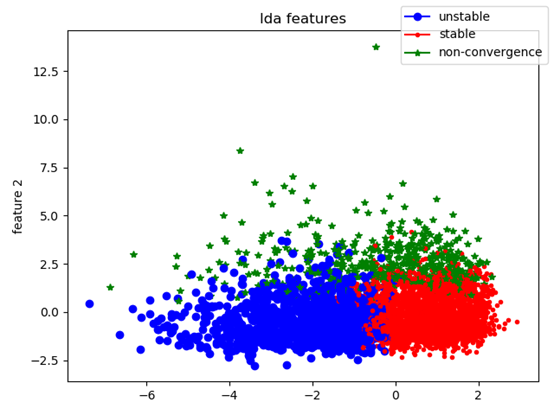可以看出三类样本在映射后的平面上分离程度较高
如果希望利用同样的模型提取新数据的主成分，则可以利用transform函数实现：
lda_feature = lda.fit_transform(test_features)#利用测试数据训练模型
lda_feature_new=lda.transform(new_data)#利用训练好的模型提取新数据的特征

展开全文机器学习
• 降维算法简介很多算法可以回归也可以分类降维算法PCA降维（主成分分析）LDA降维（线性判别分析）MDS降维（多维标度法）流形学习Isomap 简介 很多算法可以回归也可以分类 把连续值变为离散值： 1.回归模型可以做分类...深度学习 算法 监督学习
• 直观地好处是维度降低了，便于计算和可视化，其深层次的意义在于有效信息的提取综合及无用信息的摈弃，并且数据降维保留了原始数据的信息，我们就可以用降维的数据进行机器学习模型的训练和预测，但将有效提高训练和...数据分析 机器学习 算法
• 测试概述 该实验的主要目的是测试LDA（Linear Discriminant Analysis，线性...降维（PCA LDA）和训练（SVM）均是调用python的sklearn库，所有代码都在程序中给出。 每次使用相同数据集，通过三种不同方式测试，分...机器学习 SVM
• 采用PCA或LDA两种方法对数据集的数据进行降维，并进行可视化。机器学习
• 线性- LDA(Linear Discriminant ...LDA 可以提高数据分析过程中的计算效率，对于未能正则化的模型，可以降低维度灾难带来的过拟合。 LDA 降维算法展示 详细内容可参见《数据降维—线性判别分析(LDA)》： ht...算法
• 1. python实现数据降维 数据降维的情况： 1.维度数量 2.建模是否需要保留原始维度，保留：特征选择；不保留：特征转化（PCA,LDA） 3.对模型的计算效率和时效性 降维的方式：特征选择，特征转换，特征组合 import ...
• 为此需要引入有标签的线性降维学习方法LDA，其实思想是和PCA完全一致，但现在我们希望最小化类内方差，最大化类间方差。事实上这部分内容在讲线性模型时已经讲过了，我们这里复习一下。 首先定义类间距离和类内距离...数据分析 t-sne 自编码器
• LDA 既可以用于分类又可以用于降维，应用场景最多的还是降维。和 PCA 类似，LDA 降维基本也不用调参，只需要指定降维到的维数即可。 class sklearn.discriminant_analysis.LinearDiscriminantAnalysis solver=’...机器学习
• 数据降维可以降低模型的计算量并减少模型运行时间、降低噪音变量信息对于模型结果的影响、便于通过可视化方式展示归约后的维度信息并减少数据存储空间。因此，大多数情况下，当我们面临高维数据时，都需要对数据做...PCA
• 转自git: https://github.com/duoergun0729/nlp/blob/master/%E4%BD%BF%E7%94%A8LDA%E8%BF%9B%E8%A1%8C%E6%96%87%E6%A1%A3%E4%B8%BB%E9%A2%98%E5%BB%BA%E6%A8%A1.md ...LDA（Latent Dirichlet Allocation）是一种文...
• LDA模型完成，训练时间为11368.268秒 ps:主机是8g内存的，然后设置主题数目过大的时候会出现 Memroy Out 问题。在这里设置了100 #随机打印某10个文档的主题 M = 121177 num_show_topic = 10 print '...文本分类
• 一、基于特征选择的降维 基于sklearn的feature_selection进行特征选择 SelectPercentile 将变量集中的特征变量与目标变量根据指定函数进行分析打分，只保留用户指定百分比的最高得分的特征 from sklearn import ...
• ## python机器学习之特征降维

多人点赞 热门讨论 2021-06-10 13:47:56
今天我们要学的是数据的降维部分，数据的降维主要是将高维向量空间的数据点印射到低纬空间中，当然也可以从低纬度转换到高维度中，在实际应用中，高维度空间包含较多的冗余信息与噪声信息，从而机器学习 算法
• 以下内容笔记出自‘跟着迪哥学python数据分析与机器学习实战’，外加个人整理添加，仅供个人复习使用。 导入数据 import numpy as np import pandas as pd df=pd.read_csv(r'iris.data') print(df.shape) #查看...
• 图片来源于网络，文末附本文源码下载方法笔者之前写过一篇名为《用PCA方法进行数据降维》的文章，文章中主要讲述了如何用PCA（主成分分析）来对数据进行降维的方法。而今天笔者将介绍另一种常用...机器学习 人工智能 深度学习 编程语言 计算机视觉
• 购买课程后，添加小助手微信（微信号：csdn500）回复【唐宇迪】 进入学习群，获取唐宇迪老师答疑 本课程旨在帮助大家掌握机器学习中的经典算法与实战策略，从实战角度出发，通过实际的案例来讲解算法的应用与提升。...人工智能学习 深度学习框架 深度学习算法
• 关于PCA（主成分分析，Principal components analysis）,这里有非常通俗易懂的文章解释：主成份分析(PCA)最详细和全面的诠释，这里就不多啰嗦了，下面主要介绍PCA算法和LDA算法在MNIST数据集上的应用。   主要...PCA Kaggle
• 1. 采用 PCA 对男女生样本数据中的（身高、体重、鞋码、50m 成绩、肺活量） 共 5 个特征进行特征降维，并实现 LDA 算法对处理后的特征进行分类，计算 模型预测性能（包含 SE、SP、ACC 和 AUC），试分析 LDA 算法如果...机器学习
• LDA主题模型及python实现介绍了LDA模型的基本原理与Sklearn实现流程。 1 应用 聚类：主题是聚类中心，文章和多个类簇（主题）关联。聚类对整理和总结文章集合很有帮助。参看Blei教授和Lafferty教授对于Science杂志...应用
• 主成分分析的目标是向量在低维空间中的投影能有很好地近似替代原始向量，但这种投影对分类不...sklearn进行PCA及LDA分析 import matplotlib.pyplot as plt from sklearn import datasets from sklearn.discriminant_
• 1. 采用 PCA 对男女生样本数据中的（身高、体重、鞋码、50m 成绩、肺活量） 共 5 个特征进行特征降维，并实现 LDA 算法对处理后的特征进行分类，计算 模型预测性能（包含 SE、SP、ACC 和 AUC），试分析 LDA 算法如果...决策树 机器学习
• PCA降维识别手写数字 PCA 用于数据降维，减少运算时间，避免过拟合。 PCA(n_components=150,whiten=True) - n_components参数设置需要保留特征的数量，如果是小数，则表示保留特征的比例; 设为大于零的整数，会...
• python数据挖掘系列教程 PCA主成分分析 准确的PCA和概率解释： PCA 用于对一组连续正交分量中的多变量数据集进行方差最大方向的分解。 在 scikit-learn 中， PCA 被实现为一个变换对象， 通过 fit 方法可以...机器学习 数据挖掘 sklearn 算法
• 近期实验室在上一门机器学习的讨论班，第一次作业中有一道题要求实现LDA算法与QDA算法，这里LDA部分参考了以下文章与教材，详细的推导在下面的文章里也有介绍： 机器学习算法推导&手写实现03——线性判别分析 ...
• 这里有个 GitHub 项目整理了使用 Python 实现了 11 种经典的数据抽取（数据降维）算法，包括：PCA、LDA、MDS、LLE、TSNE 等，并附有相关资料、展示效果；非常适合机器学习初学者和刚刚入坑数据挖掘的小伙伴。 为......

# lda模型降维pythonpython 订阅